# Conduction: One Dimensional (Part - 6) Chemical Engineering Notes | EduRev

## Chemical Engineering : Conduction: One Dimensional (Part - 6) Chemical Engineering Notes | EduRev

The document Conduction: One Dimensional (Part - 6) Chemical Engineering Notes | EduRev is a part of the Chemical Engineering Course Heat Transfer for Engg..
All you need of Chemical Engineering at this link: Chemical Engineering

Conduction: One Dimensional, Heat Transfer

General heat conduction relation in cylindrical coordinate system (fig. 2.12) is derived (briefly) below.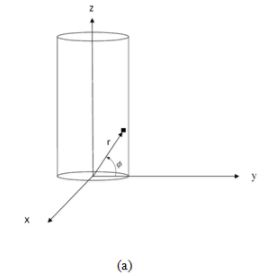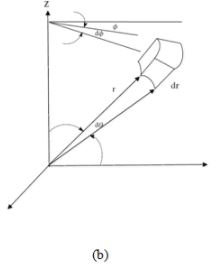Fig.2.12. Cylindrical coordinate system (a) and an element of the cylinder

The energy conservation for the system is written as,

Ӏ + ӀӀ = ӀӀӀ + ӀV     (2.21)
where,
I      : Rate of heat energy conducted in
II    : Rate of heat energy generated within the volume element
III   : Rate of heat energy conducted out
IV   : Rate of energy accumulated (ӀV)
and the above terms are defines as,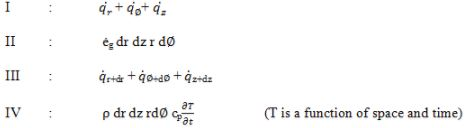Thus,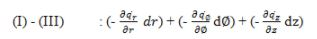###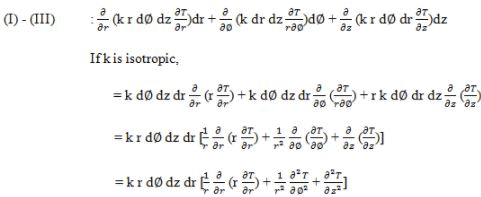On putting the values in equation 2.21,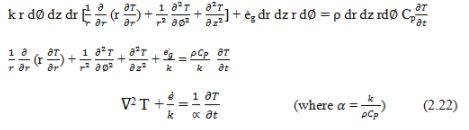Thus the Laplacian operator is,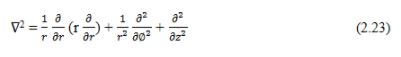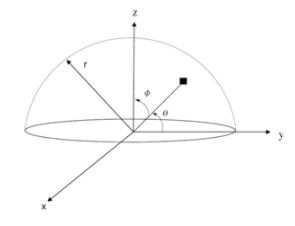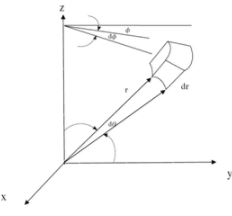(a)                                                                                         (b)

Fig.2.13. Spherical coordinate system (a) and an element of the sphere

In a similar way the general expression for the conduction heat transfer in spherical body with heat source can also be found out as per the previous discussion. The Laplacian operator for the spherical coordinate system (fig.2.13) is given below and the students are encouraged to derive the expression themselves.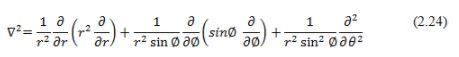Offer running on EduRev: Apply code STAYHOME200 to get INR 200 off on our premium plan EduRev Infinity!

## Heat Transfer for Engg.

46 videos|60 docs|59 tests

,

,

,

,

,

,

,

,

,

,

,

,

,

,

,

,

,

,

,

,

,

;